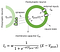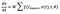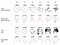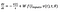# The Overlooked Side of Neural ODEs

It has been a while since I became fond of understanding what happens if the hidden state of deep neural networks is specified by ordinary differential equations (ODEs). In this article, I attempt to denote an overlooked yet paramount aspect of ODE-based neural networks to promote novel insights for future research in this direction.

From a statistical learning perspective, the concept of Neural ODEs as a new class of deep neural networks was introduced [Chen et al. 2018]. Authors suggested that instead of using a sequence of hidden layers, we can fold the hidden states, h(t), into a very deep representation, a differential equation of the form dh(t)/dt = f(h(t), t, θ), with the neural network f parametrized by θ. One can then compute the state by a chosen numerical ODE solvers, and train the network by performing reverse-mode automatic differentiation (backpropagation), either by gradient descent through the solver, or by considering the solver as a black-box and apply adjoint sensitivity method.

Neural ODEs manifest several benefits such as adaptive computations, better continuous time-series modeling, memory, and parameter efficiency.

Great! And since then, a number of alternative approaches to Neural ODEs have tried to improve their representation, use them and understand them better. Examples include Rubanova et al. NeurIPS 2019, Dupont et al. NeurIPS 2019, Durcan et al. NeurIPS 2019, Jia and Benson, NeurIPS 2019, yan et al. ICLR 2020, Holl et al. ICLR 2020, Quaglino et al. ICLR 2020.

Notwithstanding the fact that Neural ODEs provided us with an excessively powerful probabilistic modeling tool, in my personal opinion, they overlook the origin of their approximation capabilities and the primary reason why ODE-based neural models should be favorable in the first place. I will address this by a history lesson!

## Neural ODEs of the 18th Century

Let us travel back to the 18th century where the first version of ODE-based cell models was discovered primarily by the research results of the physicist Luigi Galvani, on bio-electricity. The work was followed by the discovery of action potential in nerve cells, in the 19th century by physiologist Emil du Bois-Reymond. Bois-Reymond, together with his student Julius Bernstein, developed the so-called “membrane hypothesis” based on which membrane of nerve cells act similar to an electronic capacitor (Cm) with their state being described by a voltage-current first-order differential equation. Subsequently, the first mathematical model of a neuron, termed as integrate-and-fire was introduced in 1907 by Louis Lapicque and is shown below:

The discovery of the working principles of ion channels resulted in the development of a more accurate description of dynamics of action potentials and synaptic transmission by Hodgkin and Huxley in 1952, for which they won a Nobel prize in 1963! In fact, Hodgkin and Huxley’s findings created a substrate for modeling the current I_in shown in the integrated-and-fire model above, by a more familiar expression concretely described by the early works of Christoph Koch group in 1989 in his book of Methods in Neuronal Modeling and Terry Sejnowski’s group in [Destexhe, Mainen, and Sejnowski 1994]:Synaptic current transmission from a presynaptic neuron to a postsynaptic neuron. w represents the maximum conductance of the synapse (maximum weight of the synapse), σ and μ are synaptic transmission parameters, and E stands for the reversal potential of a synapse. E determines whether a synapse is excitatory or inhibitory. Notice the sigmoidal abstraction of the synaptic transmission!

If we simplify the synaptic transmission to a conductance (Consider we only take the sigmoidal function above, as the synaptic strength, I_s ≈ f(v(t),θ)), then by substituting this synaptic conductance into Eq. 1, we have:Equation 2. Neural State of a neuron. f is the sigmoidal function shown above, and θ includes the parameters of the sigmoid function. Dejavu!

As we can see, a Neural ODE form appears by the combination of biophysical models of neurons and synapses when we simplify their notions. With this historical note, I tried to motivate the overlooked side of Neural ODEs, which is their undeniable connection to a rich set of computational models chiefly rooted in neuroscience research. I would like to finish by demonstrating how we can take advantage of this new side of Neural ODEs to build better learning systems.

## On the Expressive Power of Neural ODEs

Raghu et. al. ICML 2017 introduced novel measures of expressivity of deep neural networks unified by the notion of trajectory length. They showed that for a given input trajectory (e.g., a circular trajectory) and arbitrary initialization of the network weights, the latent trajectory length after passing through each hidden layer increases exponentially with the depth of the network and results in achieving a more complex trajectory pattern. They discovered that such representation could serve as a reliable measure for the expressivity of a given architecture.

I tried to quantify the expressivity of Neural ODEs compared to a fully connected network of the same size, from a trajectory length and shape perspective. For this purpose, I constructed six densely connected layers once with logistic sigmoid activations and once with tanh. Each layer has 100 neurons (k = 100), and all weights and biases are initialized randomly from \mathcal{N}. The neural ODE network is defined then as dh/dt = 6-layer network (Implemented by an explicit Runge-Kutta (4,5) ODE Solver), and the feedforward network as h = 6-layer network. Both networks are subjected to a 2-D circular trajectory determined by two input time-series sin(t) and cos(t) for t = [0, 2π]. Below, I plotted the 2-D projection of the trajectory transformations after each hidden layer (L1 = hidden layer 1) for FFN and neural ODE. The numbers shown over each trajectory depict their length. The increase of the trajectory length by depth does not appear for the neural ODE architecture, and its latent trajectory representation from layer to layer does not vary much. This is surprising as we do not observe a more expressive behavior from the neural ODE compared to its discretized FFN counterpart, from the trajectory length point of view! Let’s investigate this further from another perspective.Latent trajectory representation. In black, the 2D image of the transformation of the input trajectory (red circle), after each hidden layer of the networks. The numbers depict the length of the trajectory.

Back to our main point, when we constructed the model for the neural ODE from biologically plausible neural and synaptic models, we simplified their models dramatically in order to show they can express a neural ODE- like semantics. Now, what if we do not perform the simplifications and try to represent the neurons’ equations closer to that of natural learning systems, and deploy the synaptic transmission current in full? Would we get a more expressive learning system? Let’s try this! One of the simplest representations of a neuron’s dynamics is presented in Eq. 3, where the neuron possesses a leakage compartment (-v(t)/τ), to stabilize its autonomous behavior (when there are no inputs to the cell) as follows:Equation 3. A leaky membrane integrator model, or from a machine learning perspective, a continuous-time recurrent neural network (CT-RNN) [Funahashi et al. 1993]. τ determines the speed at which a neuron approaches its resting state.

Now, if we plug in the full synaptic current I_s formula, into this equation and write it in a canonical form, we get:Equation 4. A liquid time-constant network (LTC). A neural model with varying time-constant. Denote the neural network instance appearance in both the time-constant part of the ODE and the state of the ODE. Is this version of a neural ODE more expressive? Let’s see!

Now let us perform the trajectory space expressivity analysis on this evolved neural ODE model. In the following, I constructed a single-layer neural ODE (Eq. 2), a CT-RNN (Eq. 3) and an LTC network (Eq. 4), with different width (number of neurons, k = {10, 25, 100}), and for three different activation functions, ReLU, Logistic sigmoid and tanh. Then, we computed the latent trajectory space of the hidden layer when it is exposed to a circular trajectory, as described in the previous experiment. Below, we see instances of the latent trajectory representation of these variants of neural ODEs, when the weights and biases of the networks are set randomly from Gaussian distributions N(0,σ² = 2), and N(0,σ² =1), respectively.

As we observe, vanilla Neural ODE and CT-RNN architectures do not change their latent representations, while the LTC network realizes a series of complex patterns. Moreover, we see that the trajectory length (stated over each sub-figure), is exponentially growing for the LTC networks by increasing the network’s size. The complexity of the generated patterns by the LTC network is a demonstration of their expressive power in realizing a variform of dynamics given a simple input trajectory. At the same time, we cannot expect such creative complexity from the Neural ODE architectures.

To conclude, I tried to motivate an overlooked side of neural ODEs, which is their close connection to the computational elements of natural learning systems. Additionally, I showed how a more biophysically realistic variant of Neural ODEs, the liquid time-constant (LTC) networks, give rise to a much more expressive representation.

The next question is, how would networks of LTC neurons perform as a learning system, in real-life applications? Can they serve as a more expressive learning system compared to state-of-the-art deep learning methods? Some very preliminary results on this are provided in our work Lechner et. al. ICRA 2019, and a lot more on the mathematical side of time-continuous neural networks will be made available in a couple of days in my PhD thesis! In the meantime feel free to watch TEDx talks on this topic:

TEDxVienna 2018

TEDxCluj 2019

## Analytics Vidhya

Analytics Vidhya is a community of Analytics and Data…

## Analytics Vidhya

Analytics Vidhya is a community of Analytics and Data Science professionals. We are building the next-gen data science ecosystem https://www.analyticsvidhya.com

Written by

## Ramin Hasani

Machine Learning Scientist## Analytics Vidhya

Analytics Vidhya is a community of Analytics and Data Science professionals. We are building the next-gen data science ecosystem https://www.analyticsvidhya.com